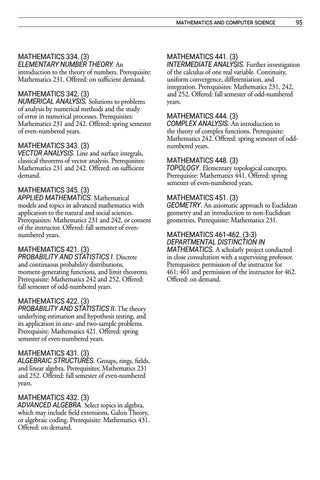mathematics and computer science

MATHEMATICS 334. (3) ELEMENTARY NUMBER THEORY. An introduction to the theory of numbers. Prerequisite: Mathematics 231. Offered: on sufficient demand. MATHEMATICS 342. (3) NUMERICAL ANALYSIS. Solutions to problems of analysis by numerical methods and the study of error in numerical processes. Prerequisites: Mathematics 231 and 242. Offered: spring semester of even-numbered years. MATHEMATICS 343. (3) VECTOR ANALYSIS. Line and surface integrals, classical theorems of vector analysis. Prerequisites: Mathematics 231 and 242. Offered: on sufficient demand. MATHEMATICS 345. (3) APPLIED MATHEMATICS. Mathematical models and topics in advanced mathematics with application to the natural and social sciences. Prerequisites: Mathematics 231 and 242, or consent of the instructor. Offered: fall semester of evennumbered years. MATHEMATICS 421. (3) PROBABILITY AND STATISTICS I. Discrete and continuous probability distributions, moment-generating functions, and limit theorems. Prerequisite: Mathematics 242 and 252. Offered: fall semester of odd-numbered years. MATHEMATICS 422. (3) PROBABILITY AND STATISTICS II. The theory underlying estimation and hypothesis testing, and its application in one- and two-sample problems. Prerequisite: Mathematics 421. Offered: spring semester of even-numbered years. MATHEMATICS 431. (3) ALGEBRAIC STRUCTURES. Groups, rings, fields, and linear algebra. Prerequisites: Mathematics 231 and 252. Offered: fall semester of even-numbered years. MATHEMATICS 432. (3) ADVANCED ALGEBRA. Select topics in algebra, which may include field extensions, Galois Theory, or algebraic coding. Prerequisite: Mathematics 431. Offered: on demand.

95

MATHEMATICS 441. (3) INTERMEDIATE ANALYSIS. Further investigation of the calculus of one real variable. Continuity, uniform convergence, differentiation, and integration. Prerequisites: Mathematics 231, 242, and 252. Offered: fall semester of odd-numbered years. MATHEMATICS 444. (3) COMPLEX ANALYSIS. An introduction to the theory of complex functions. Prerequisite: Mathematics 242. Offered: spring semester of oddnumbered years. MATHEMATICS 448. (3) TOPOLOGY. Elementary topological concepts. Prerequisite: Mathematics 441. Offered: spring semester of even-numbered years. MATHEMATICS 451. (3) GEOMETRY. An axiomatic approach to Euclidean geometry and an introduction to non-Euclidean geometries. Prerequisite: Mathematics 231. MATHEMATICS 461-462. (3-3) DEPARTMENTAL DISTINCTION IN MATHEMATICS. A scholarly project conducted in close consultation with a supervising professor. Prerequisites: permission of the instructor for 461; 461 and permission of the instructor for 462. Offered: on demand.